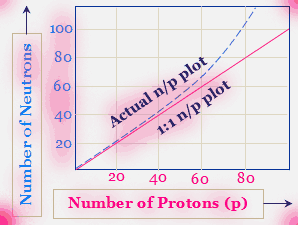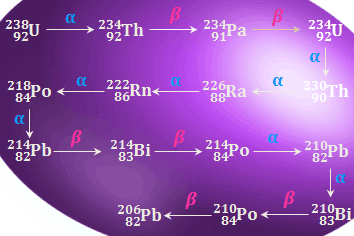Pairing of the nuclear spins

Since radioactivity is a nuclear phenomenon it must be connected with the instability of the nucleus. We know that the nucleus of an atom is composed of two fundamental particles, protons, and neutrons. Since all elements are not radioactive, the ratio of the neutron to the proton of the unstable, radioactive nucleus is the factor responsible for radioactivity.
Nuclear scientists studied this problem and concluded that stability or instability is connected with the pairing of the nuclear spins.
Just as electrons spin around their own axes and just as electron- spin pairing leads to be stable chemical bonds, so also the nuclear protons and neutrons spin around their own axes, and pairing of spins of neutrons among neutrons, and pairing of spins of protons among protons leads to nuclear stability. When all the non-radioactive, stable isotopes of elements are considered it is observed that,
1. Nuclei with an even number of protons and even number of neutrons are most abundant and most stable. It is observed that even number leads to spin pairing, and odd number leads to unpaired spins.
2. Nuclei with an even number of neutrons and odd number protons or an odd number of neutrons and even number of protons slightly less stable than even number of neutrons and protons.
3. By far the least stable isotopes are those which have odd numbers of protons and an odd number of neutrons. The nuclear spin pairing is a maximum when odd numbers of both are present.

Protons and neutrons ratio

Along with the odd or even number of protons and neutrons, nuclear stability also influenced by the relative numbers of protons and neutrons.
The neutron/proton ratio (n/p) helps us to predict which way an unstable radioactive nucleus will decay.Protons to neutrons are the cause of radioactivity
The above graph is obtained by plotting the number of neutrons in the nuclei of the stable isotopes against the respective number of protons.
A study of this figure shows that the actual n/p plot of stable isotopes breaks from hypothetical 1:1 plot around an atomic number 20, and thereafter rises rather steeply, this indicates that as the number of protons increases inside the nucleus more and more neutrons are needed to minimize the proton-proton repulsion and thereby to add to nuclear stability.
Neutrons, therefore, serve as binding martial inside the nucleus. The way an unstable nucleus disintegrates will be resided by its position with respect to the actual n/p plot of nuclei.
When the isotope located above this actual n/p plot it is too high an n/p ratio, and when it is located below the plot it is too low in n/p ratio. In either case, the unstable nucleus should decay so as to approach the actual n/p plot. We now discuss the two cases of decay.

Neutron- to - proton ratio too high

Isotopes with too many neutrons in the nucleus ( that is, with more neutrons than its needs for stability) can attain greater nuclear stability if one of the decays of the neutron to the proton. Such a disintegration leads to the ejection of an electron from inside the nucleus.
0n1 1H1 + -1n0(electron)
Thus beta ray emission will occur whenever the n/p ratio is higher than the n/p value expected for stability.
This is almost always the case when the mass number of radioactive isotopes is greater than the average atomic weight of the element.

Neutron- to - Proton ratio too low

A nucleus deficient in neutrons will tend to attain nuclear stability by converting one of its protons to a neutron and this will be achieved either by the emission of a position or by the capture of an electron.
1H1 0n1 + +1e0(Positron)
Such Decay occurs with a radioactive isotope whose mass number is less than the average atomic weight of the element. Positron emission occurs with light isotopes of the elements of low atomic number.
 7N13  → 6C13 + +1e0 53I121  → 52Te121 + +1e0
Orbital electron capture occurs with too light isotopes ( too low n/p) of the elements of relatively high atomic numbers. For such elements, the nucleus captures an electron from the nearest orbital ( K shell; n = 1) and thus changes one of its protons to a neutron.
 37Rb82 + -1e0 → 36Kr82 79Au194 + -1e0 → 78Pt194
Among the heaviest nuclei, the total proton-proton repulsion is so large that the binding effect of the neutron is not enough to lead to a stable nonradioactive isotope. For such nuclei, alpha particle emission is the common mode of decay.
 90Th232 → 88Ra228 + 2He4 92U235 → 90Th231 + 2He4 92U238 → 90Th234 + 2He4

Units of radioactivity measurement

In Practice, radioactivity is expressed in terms of the number of disintegration per second. One gram of Radium undergoes about 3.7 × 10¹⁰ disintegrations per second.
The quantity of 3.7 × 10¹⁰ disintegrations per second is called curie, which is the older unit of radioactivity. Millicurie and microcurie respectively correspond to 3.7×10⁷ and 3.7×10⁴ disintegration per second.
• Explanation:
On this basis, the radioactivity of radium is 1 curie per gram. Phosphorus-32, a beta - emitter, has an activity of 50 millicuries per gram. This means that for every gram of phosphorus-32 in some material containing this species, there are 50 × 3.7 × 10⁷ disintegrations taking place per second.
1 disintegration per second is called becquerel(Bq), it is the S.I. unit of radioactivity.
 1 Curie = 3.7 × 10¹⁰ Bq
Another practical unit of radioactivity is Rutherford(Rd).

When an Alpha particle ejected from within the nucleus the mother element loss two units of atomic number and four units of mass number. Thus, if a radioactive element with mass number M and atomic number Z ejected an alpha particle the newborn element has mass number = (M - 4) and atomic number = (Z - 2).
₈₈Ra²²⁶ ₈₈₋₂Rn²²²⁻⁴ + ₂He⁴(∝) ₈₈Ra²²⁶ ₈₈Rn²²² + ₂He⁴(∝)
When a beta particle is emitted from the nucleus, the daughter element nucleus has an atomic number one unit greater than that of the mother element nucleus. Thus, if a radioactive element with mass number M and atomic number Z ejected a beta particle the newborn element has mass number the same and atomic number = (Z + 1).
₉₀Th²³⁴ ₉₁Pa²³⁴ + ₋₁e⁰(β)

Disintegration series

We have just seen that the radioactive elements continue to undergo successive disintegration till the daughter elements become stable, non- radioactive isotopes of lead. The mother element along with all the daughter elements down to the stable isotope of lead is called a radioactive disintegration series.

Uranium - 238 disintegration series

Uranium - 238 decays ultimately to an isotope of lead. The entire route involves alpha emissions in eight stages and beta emission in six stages, the overall process being,
₉₂U²³⁸ ₈₂Pb²⁰⁶ + 8 ∝ + 6 β
The mass number of all the above disintegration products are given by (4n +2) where n = 59 for Uranium - 238. This disintegration series is known as (4n + 2) series.Uranium - 238 radioactive series in cause of radioactivity

Uranium - 235 disintegration series

The (4n+3) series (n=an integer) starts with Uranium - 235(n=58) and end with the stable isotope Lead - 207(n=51).
The entire route involves alpha emissions in seven stages and beta emission in four stages, the overall process being,
₉₂U²³⁵ ₈₂Pb²⁰⁷ + 7 ∝ + 4 βUranium - 235 radioactive series in the cause of radioactivity

Thorium- 232 disintegration series

The (4n) series (n=an integer) starts with Thorium - 232(n=58) and end with the stable isotope Lead - 208(n=52).
The entire route involves alpha emissions in six stages and beta emission in four stages, the overall process being,
₉₀Th²³² ₈₂Pb²⁰⁶ + 6 ∝ + 4 βThorium - 232 radioactive series in the cause of radioactivity

Group displacement law

When an alpha particle is emitted in a radioactive disintegration step, the product is displaced two places to the left in the Periodic Table but the emission of a beta particle results in a displacement of the product to one place to the right.
Problem
79Au197 is nonradioactive but 88Ra226 is Radioactive-Why?
The number of the neutron in gold(Au) is 118 and proton is 79. So, n/p ratio = 118/79 =1.49 Which is less than 1.5 thus Au-197 is stable.
But in Ra - 226 the number of Neutrons(n) = 138 and number of proton(p) = 88. So n/p ratio = 1.57. Thus, Ra-226 is radioactive.
Problem
Which of the following elements are beta emitter and why? (i) 6C12 and 6C14 (ii) 53I127 and 53I133.
1. The n/p ratio for stable carbon C-12 is 1.0(6n + 6p) but that for C - 14 is 1.3 (8n + 6p). It will predict that carbon -14 will be radioactive and will emit beta rays.
• 6C14 7N14 + -1e0( beta ray)
2. Similarly the n/p ratio of the stable Iodine-127 is 1.4 (74n + 53p). For Iodine-133 the n/p ratio equals 1.5 (80n + 53p) and it is again predicted to the beta emitter.
53I127 54Xe137 + -1e0(beta ray)
Problem
If a radioactive element x number disintegration per second. Express the radioactivity of this element in Curie.
3.7 × 10¹⁰ disintegrations per second = 1 curie .
Thus x number of disintegration per second = x/(3.7 × 10¹⁰) Curie.

Cause of radioactivity, Protons and Neutrons ratio, Units of radioactivity measurement, Radioactive Disintegration Series and Group displacement Low